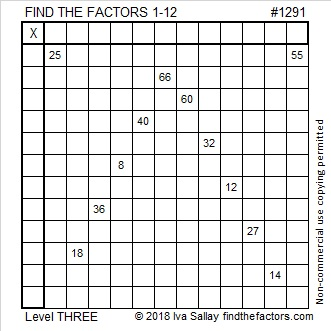# 1291 and Level 3

If you think of the common factors of 25 and 55, then you have actually started to solve this puzzle! Start at the top of the puzzle and work your way down. Try it!Print the puzzles or type the solution in this excel file: 12 factors 1289-1299

Here are some facts about the number 1291

• 1291 is a prime number.
• Prime factorization: 1291 is prime.
• The exponent of prime number 1291 is 1. Adding 1 to that exponent we get (1 + 1) = 2. Therefore 1291 has exactly 2 factors.
• Factors of 1291: 1, 1291
• Factor pairs: 1291 = 1 × 1291
• 1291 has no square factors that allow its square root to be simplified. √1291 ≈ 35.93049

How do we know that 1291 is a prime number? If 1291 were not a prime number, then it would be divisible by at least one prime number less than or equal to √1291 ≈ 35.9. Since 1291 cannot be divided evenly by 2, 3, 5, 7, 11, 13, 17, 19, 23, 29 or 31, we know that 1291 is a prime number.1291 is also palindrome 1D1 in BASE 30 because 30² + 13(30) + 1 = 1291

This site uses Akismet to reduce spam. Learn how your comment data is processed.